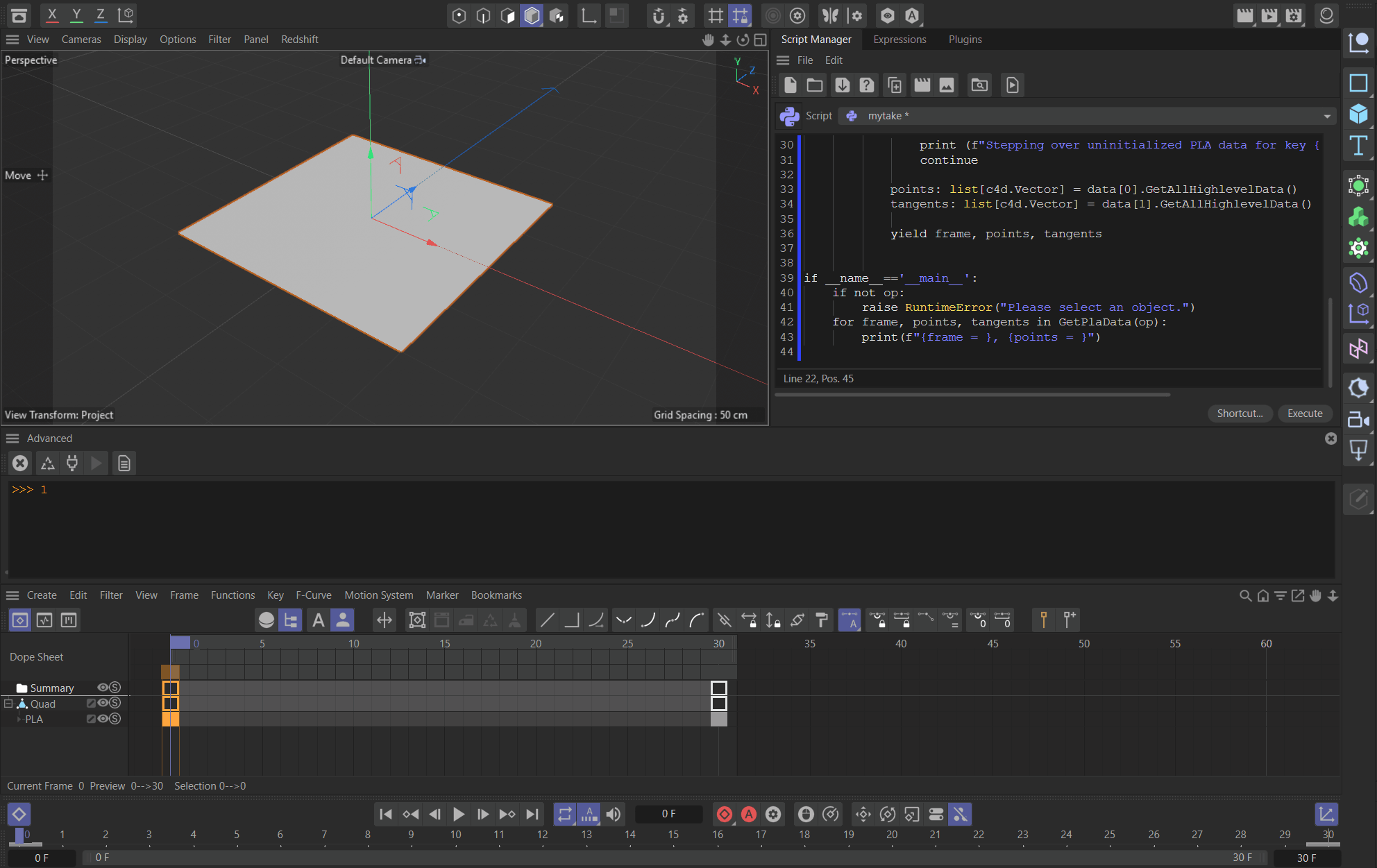# UNSOLVEDGet Spline Points Positions from PLA keyframes

Hi,

I am tasked with obtaining the position of spline points from the PLA keyframes of the set spline.

From the SDK, I ended up at a `<c4d.PLAData object at 0x000001B118B7F2C0>`
But I do not know how to extract the spline points' positions from this PLAData object.

Code to get the PLAData object:

``````import c4d
spline = doc.SearchObject('Spline')
track = None
PLA = c4d.DescID(c4d.DescLevel(c4d.CTpla, c4d.CTpla))
tracks = spline.GetCTracks()

for t in tracks:
tid = t.GetDescriptionID()
if tid == PLA : track = t

curve = track.GetCurve()
for i in range(curve.GetKeyCount()):
key = curve.GetKey(i)
data = key.GetGeData()
print(data[c4d.CK_PLA_DATA])

def main():

if __name__=='__main__':
main()
``````

I also tried setting the document time with `doc.SetTime(key.GetTime())` and then `spline.GetAllPoints()` but I am getting the same points' positions all the time.

What am I doing wrong? Or how can I get the points' positions from the PLAData Object?

Hey @joel,

Thank you for reaching out to us. You almost got itYou must unpack the c4d.PLAData which consists of a `PointTag` and a `TangentTag`. These hold then the raw data, but tangents are only yielded for spline objects. Find an example below.

Cheers,
Ferdinand

Result:``````frame = 0, points = [Vector(-100, 0, -100), Vector(-100, 0, 100), Vector(100, 0, -100), Vector(100, 0, 100)]
frame = 30, points = [Vector(0, 0, -141.421), Vector(-141.421, 0, 0), Vector(141.421, 0, 0), Vector(0, 0, 141.421)]
``````

Code:

``````"""Showcases how to access the animation data of a PLA track.
"""

import c4d
import typing

op: typing.Optional[c4d.BaseObject] # The currently selected object, can be None.

tuple[int, list[c4d.Vector], list[tuple[c4d.Vector, c4d.Vector]], None, None]]:
"""Yields all PLA data of #node as triples in the form (frame, points, tangents).

Meaningful tangent data will only be yielded for spline objects.
"""
# Input validation.
if not isinstance(node, c4d.PointObject):
raise TypeError(f"{node = }")

doc: typing.Optional[c4d.documents.BaseDocument] = node.GetDocument()
if not isinstance(doc, c4d.documents.BaseDocument):
raise IOError(f"{node} is not attached to a document.")

# Check what kind of point object #node is.
isSpline: bool = isinstance(node, c4d.SplineObject)

# Iterate over all tracks.
for track in node.GetCTracks():
if track.GetType() != c4d.CTpla:
continue

# This is a PLA track, iterate over its keys.
curve: c4d.CCurve = track.GetCurve()
for kid in range(curve.GetKeyCount()):
key: c4d.CKey = curve.GetKey(kid)
frame: int = key.GetTime().GetFrame(doc.GetFps())

# Get the PLA data and unpack it into the point and tangent tag storing the data.
if not data or len(data) != 2:
print (f"Stepping over uninitialized PLA data for key {kid} in {node}.")
continue

# Both PointTag and TangentTag are instances of VariableTag, i.e., we can access their
# data with VariableTag::GetAllHighlevelData(). We could also use low level access, but
# it does not yield any benefits here. For point objects which are not splines, we
# return the empty list for the tangents.
points: list[c4d.Vector] = data.GetAllHighlevelData()
tangents: list[c4d.Vector] = data.GetAllHighlevelData() if isSpline else []

# Yield the data.
yield frame, points, tangents

if __name__=='__main__':
if not op:

# Iterate over all data points in the PLA animations of #op.
for frame, points, _ in GetPlaData(op):
print(f"{frame = } - {points = }")
``````

I am trying your code however I am getting `ReferenceError: the object 'c4d.PointTag' is not alive`.
What could be the issue?

Hey @joel,

a 'dead' object means that Python tries to reference a node which does not exist in the C++ layer anymore, i.e., has been de- or reallocated. Since my function retrieves the tag on the spot and does not attempt to pass it outside of the function, it seems a bit unlikely that the tag is already dead, at least I am not experiencing any of this.

Please provide the scene, code you are running, and the exact stack trace (do not forget to save your script first, so that the trace has the correct line numbers). Otherwise I will not be able to help you.

Cheers,
Ferdinand